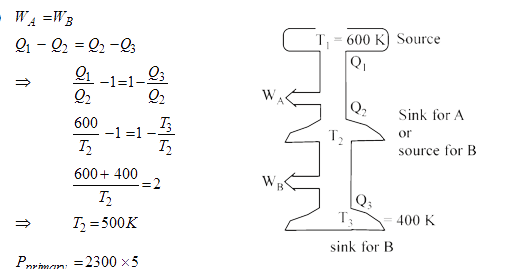# Two Carnot engines A and B are operated in series. The first one, A, receives heat at T1(=600K) and rejects to a reservoir at temperature T2. The second engine and, in turn, rejects to a heat reservoir at T3(=400K) Calculate the temperature T2 (in K) if the work outputs of the two engines are equal.

##### Updated On: 27-06-2022

लिखित उत्तर

The temperature T2 (in K) if the work outputs of the two engines are equal.Edit